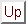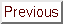Next: The basic trust-region algorithm Up: An introduction to the Previous: Trust Region and Line-search   Contents

# A simple trust-region algorithm.

In all trust-region algorithms, we always choose. The length of the steps will be adjusted using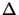, the trust region radius.
Recall that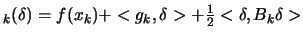is the quadratical approximation of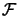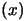around.
A simple trust-region algorithm is:
1. solve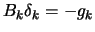subject to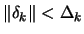.
2. Compute the degree of agreement''betweenand: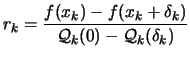(2.15)

3. updateand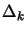: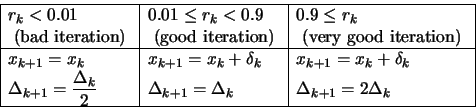(2.16)

4. Increment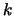. Stop if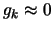otherwise, go to step 1.

The main idea in this update is: only increasewhen the local approximatorreflects well the real function.
At each iteration of the algorithm, we need to have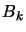and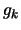to compute.
There are different ways for obtaining:
• Ask the user to provide a function which computes explicitly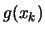. The analytic form of the function to optimize should be known in order to be able to derivate it.
• Use an Automatic differentiation tool'' (like ODYSSEE''). These tools take, as input, the (fortran) code of the objective function and generate, as output, the (fortran) code which computes the function AND the derivatives of the function. The generated code is called the adjoint code''. Usually this approach is very efficient in terms of CPU consumption.
If the time needed for one evaluation ofis 1 hour, than the evaluation of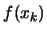ANDusing the adjoint code will take at most 3 hours (independently of the value of: the dimension of the space). This result is very remarkable. One drawback is the memory consumption of such methods which is very high. For example, this limitation prevents to use such tools in domain of Computational Fluid Dynamics'' code.
• Compute the derivatives of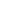using forward finite differences:(2.17)

If the time needed for one evaluation ofis 1 hour, then the evaluation ofANDusing this formulae will take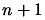hours. This is indeed very bad. One advantage, is, if we haveCPU's available, we can distribute the computing load easily and obtain the results in 1 hour.
One major drawback is that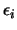must be a very small number in order to approximate correctly the gradient. If there is a noise (even a small one) on the function evaluation, there is a high risk thatwill be completely un-useful.
• Extract the derivatives from a (quadratic) polynomial which interpolates the function at points close to. This approach has been chosen for CONDOR.
When there is noise on the objective function, we must choose the interpolation sites very carefully. If we take points too close from each other, we will get a poor model: it's destroyed by the noise. If we take points very far from each other, we don't have enough information about the local shape of the objective function to guide correctly the search.
Beside, we need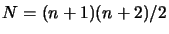points to build a quadratic polynomial. We cannot computepoints at each iteration of the algorithm. We will see in chapter 3 how to cope with all these difficulties.
There are different ways for obtaining. Many are unpractical. Here are some reasonable ones:
• Use a BFGS-update''. This update scheme uses the gradient computed at each iteration to progressively construct the Hessian matrix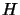of. Initially, we set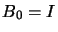(the identity matrix). If the objective function is quadratic, we will have afterupdate,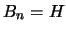exactly (Sinceis a quadratic polynomial,is constant over the whole space). If the objective function is not a quadratical polynomial,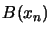is constructed using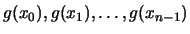and is thus a mixture of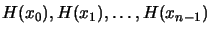. This can lead to poor approximation of, especially if the curvature is changing fast.
Another drawback is thatwill always be positive definite. This is very useful if we are using Line-search techniques but is not appropriate in the case of trust-region method. In fact,can be a very poor approximation of the real shape of the objective function if, locally,is indefinite or is negative definite. This can lead to a poor search direction.
If there is noise on the objective function and if we are using a finite difference approximation of the gradient, we will get a poor estimate of the gradient of the objective function. Sinceis constructed using only the gradients, it will also be a very poor estimate of.
• Extractfrom a (quadratic) polynomial which interpolates the function at points close to. This approach has been chosen in CONDOR.
The point are chosen close to.is thus never perturbed by old, far evaluations.
The points are far from each others'' to be less sensitive to the noise on the objective function.can also be positive, negative definite or indefinite. It reflects exactly the actual shape of.Next: The basic trust-region algorithm Up: An introduction to the Previous: Trust Region and Line-search   Contents
Frank Vanden Berghen 2004-04-19